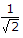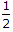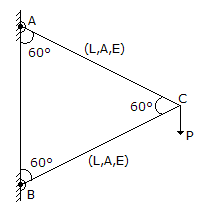# Civil Engineering - UPSC Civil Service Exam Questions

6.
A beam of square section of side 'a' is plane in the following way :
Case I: with two sides horizontal
Case II: with a diagonal horizontal
The ratio of the section modulus of Case I that of Case II is
2
2Explanation:
No answer description is available. Let's discuss.

7.
Consider the following statements :
1. Tests on cement paste to determine initial and final setting times are done at normal consistency condition.
2. Low heat cement has a high percentage to tricalcium aluminate.
3. High early strength portland cement contains a larger percentage of tricalcium silicate and a lower percentage of dicalcium silicate.
Which of these statements are correct ?
1 and 2
1 and 3
2 and 3
1, 2 and 3
Explanation:
No answer description is available. Let's discuss.

8.
What is the vertical deflection of joint C of the frame shown in figure ?PL/AE
2PL/AE
PL/2AE
3PL/AE
Explanation:
No answer description is available. Let's discuss.

9.
Which of the following pairs is not correctly matched ?
B.O.D. - Strength of sewage
Methane - Product of anerobic decomposition
Nitrate - Methemoglobinemia